# Logistic Regression Introduction

## Logistic regression

Logistic regression is a supervised learning process, where it is primarily used to solve classification problems. Unlike Linear Regression, where the model returns an absolute value, Logistic regression returns a categorical value. Here, in this series of tutorials, you will learn about Multivariate Logistic regression.

Below are a few examples, where we need answers categorically not in absolute number.

-Is the person suffering from diabetes? Yes/True or No/False.

-Will the employee leave the company? Yes/True or No/False.

-Should the applicant be granted a loan or not?

-Will the customer pay the bill or not?

-Predict the mail is spam or ham?

Classification can be of two types.

1. Binary classification
2. Multinomial classification

While the problem has two possible outcomes, then this is called Binary Classification whereas more than two possible outcomes are called multinomial classification.

For example, A person suffering from diabetes is a binary classification problem as the outcome is either Yes or No. However, the classification of a handwritten digit is an example of multinomial classification.

Let’s use the diabetes dataset to understand logistic regression.

 Blood Sugar Level Diabetes 190 0 240 1 300 1 160 0 200 1 269 1 129 0 141 0 220 0 337 1

where

0 – No

1 – Yes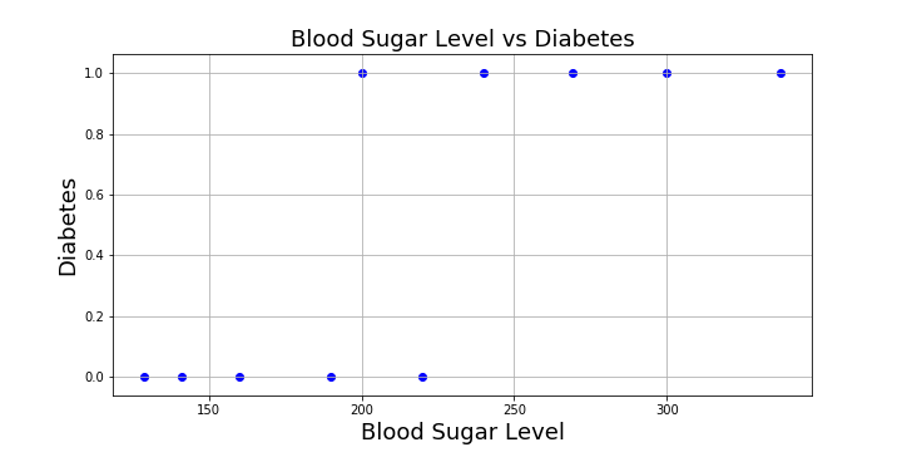Based on the graph, you can segregate Diabetes and Non-Diabetes like below.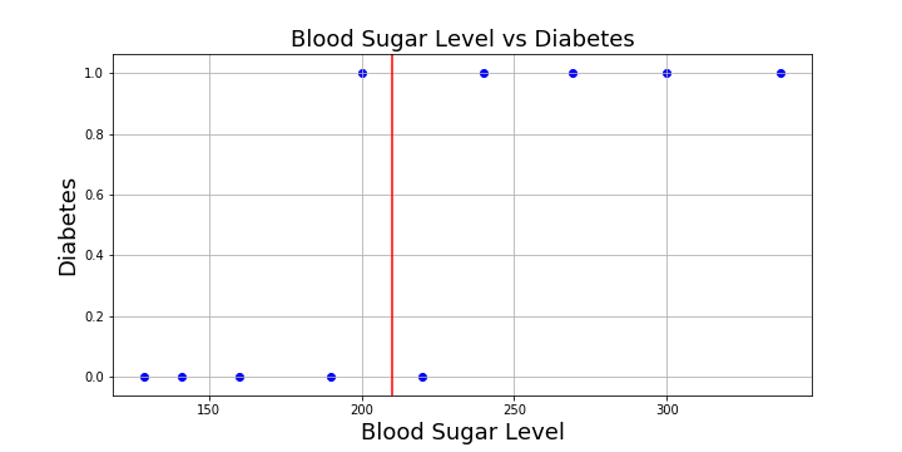Now based on the boundary, you can notice 2 data points do not classify correctly. Straight-line fit is not the right choice here.

Sigmoid Curve:

Instead of a straight-line, let us fit a curve like below. This is called a sigmoid curve.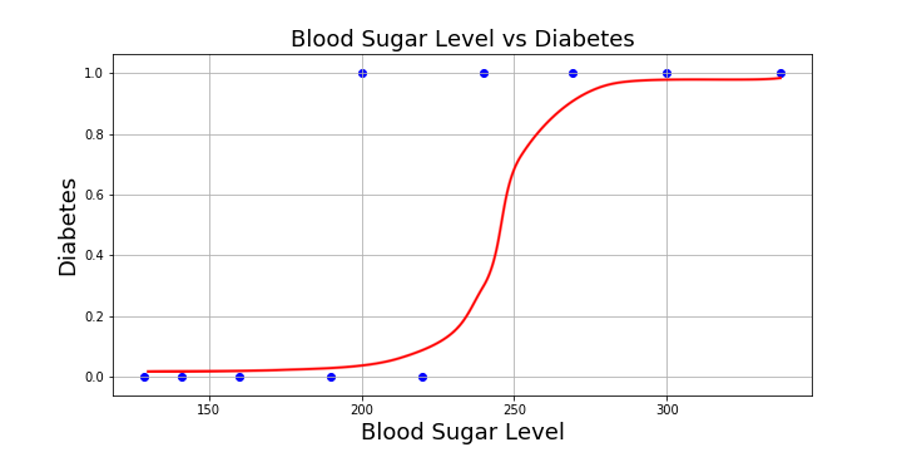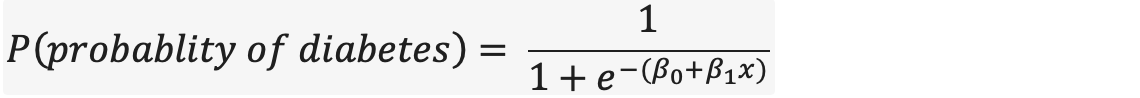Let’s think in terms of probability where the probability of having diabetes is very low (near to 0) where blood sugar level is very low (less than 150) whereas the probability of having diabetes is very high (near to 1) where blood sugar level is very high. Similarly, the probability of an intermediate blood sugar level can be around 0.4 to 0.7.

However, there may be a question of why sigmoid if we can fit a straight line as shown below.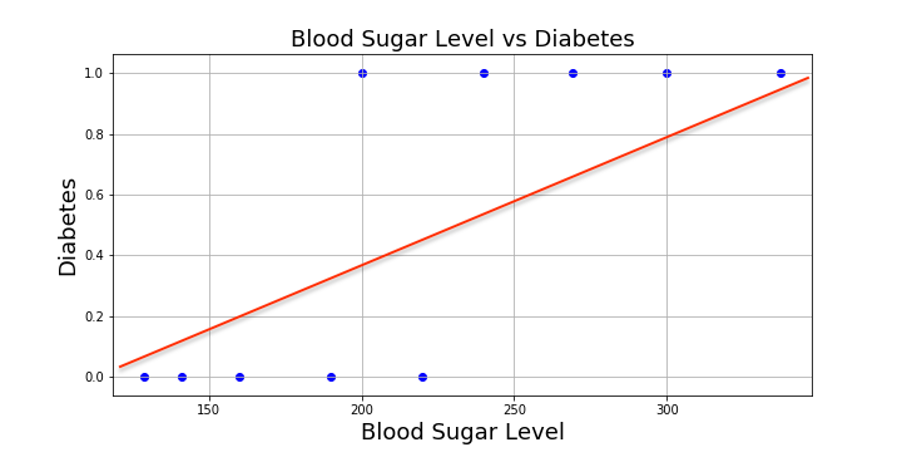Here the straight line is uniform in nature where diabetes is linearly dependent upon the Blood sugar level. But Diabetes is something dependent based upon a certain range of blood sugar levels. The probability of diabetes increases suddenly after a certain range of sugar levels not uniformly.

Multivariate Logistic regression:

The above sigmoid equation is called univariate logistic regression as it depends upon only one attribute (Blood Sugar Level). In real-time, the dependent variable can be much more than just one attribute. In such a case, the logistic regression will be like below.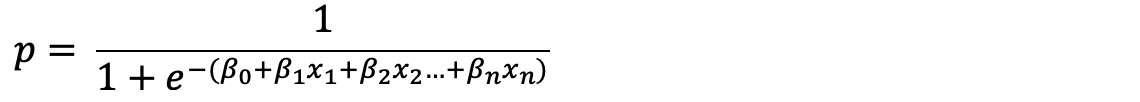Now the question is which is a good fit sigmoid curve. Let’s understand this in the next chapter.